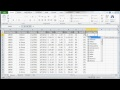## Excel Tutorial 7 of 15 – Using the IF FormulaMicrosoft Excel Tutorial 7 of 15. How to use the IF formula in Excel. The IF formula in Microsoft Excel can be used to see if a cell meets certain criteria, …

Don’t forget to check out our other video tutorials or share this video with a friend.0 votesLoading... 1,860 views

### 7 responses to “Excel Tutorial 7 of 15 – Using the IF Formula”

1.Hemant Giri says:

u teach so nice and easy way thank u so much ﻿

2.Ivan Carrillo says:

do I need if I have a salesforce list that I want to automatically update
its order based on sales amount also in excel? ﻿

3.Don Flood says:

Great tutorial; will use with my students. Thank you.

4.Snowyscar says:

Great tutorials! What you should do is post a link to an uploaded document
with the same characteristics as the one you used in your videos, and let
people try to do what you did. People learn best when combined with
practice.

5.robertbobm says:

=IF(A1=1,5000,IF(A1=2,4000,IF(­A1=3,3000,”no match”))) This says: if a1 =
1, then put 5000, if a1 = 2 then put 4000, if a1 = 3 then put﻿ 3000,
otherwise put “no match”.

6.evilpisces says:

Thank you, all these tutorials are very good, clear and helpful.

7.robertbobm says:

Thanks! Yes, that’s certainly possible. There are a couple of different
ways to accomplish this. One way would﻿ be what you’re referring to, which
is called a “nested IF” statement, where you put an IF statement inside
another IF statement. Youtube doesn’t let me use “greater than” symbols in
comments here, so I can’t use your example, but here’s a similar example: